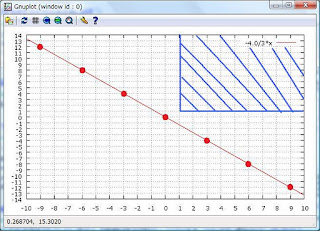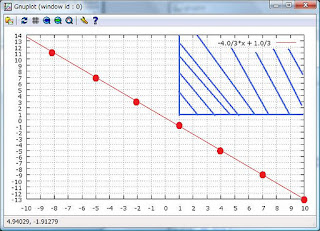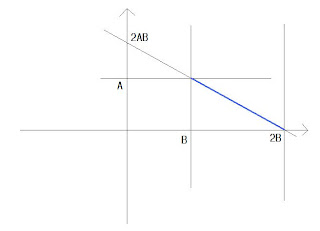## 2011年10月4日火曜日

### What numbers can Ax + By represent?

It's well known that, when two integers A and B are given, N can be represented as Ax + By when N can be dividable by (A, B). So when A and B are relatively prime, that is (A, B) = 1, All the integers can be represented as Ax + By for some integer (x, y).

But what if when a restriction is given that x and y are positive integers?
For instance, let's consider (A, B) = (4, 3). Since two numbers are co-prime, you can represent 1 as 4 * (-2) + 3 * 3 = 1. But here y is a negative integer. Other than (x, y) = (-2, 3), many other candidate solutions will hit your mind-- (-11,15), (-8,11), (-5,7), (1,-1), (4,-5), (7,-9), and so forth--. But all of them have a negative integer.

In general, the following holds:
When A and B are relatively prime, A x + B y can represent all the numbers above and equal to 2AB for some positive integers (x, y).

The following graphs are self-explainary.
The first one is plotting 4x+3y = 0. The second 4x+3y = 1, the third 4x+3y=11, a case where a feasible solution exists. And the last one shows why Ax + By can represent all the numbers over 2AB.